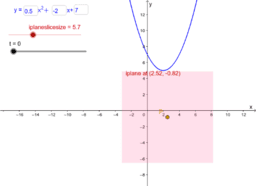Complex points using iplanes

You can visualise where points with complex coordinates are using iplanes, which is described starting at this blog post: http://blogs.adelaide.edu.au/maths-learning/2016/08/05/where-the-complex-points-are/ These GeoGebra activities will allow you to investigate the concepts.
•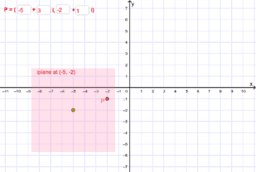Complex point locator

•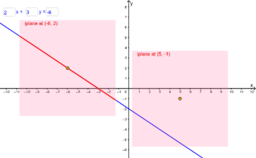Complex points on a real line

•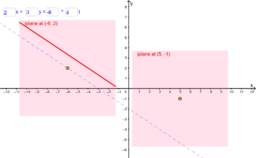Complex line with real slope

•Complex line with unreal slope

•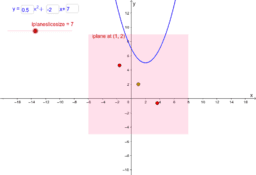Complex points on a parabola

•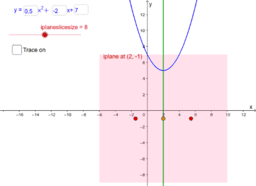Complex points on a parabola - iplanes on a vertical line

•